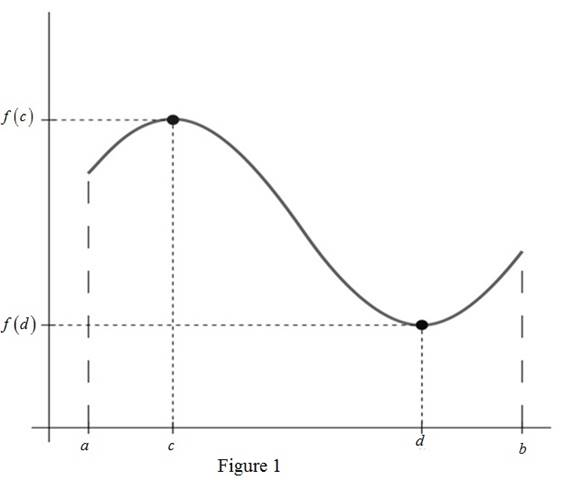# The Extreme value theorem.### Single Variable Calculus: Concepts...

4th Edition
James Stewart
Publisher: Cengage Learning
ISBN: 9781337687805### Single Variable Calculus: Concepts...

4th Edition
James Stewart
Publisher: Cengage Learning
ISBN: 9781337687805

#### Solutions

Chapter 4, Problem 2RCC

(a)

To determine

## To explain: The Extreme value theorem.

Expert Solution

### Explanation of Solution

The extreme value theorem is stated as follow.

Extreme Value Theorem:

“If f is a continuous function on [a,b] then f attains its absolute maximum value f(c) and absolute minimum value f(d) at some points c and d in the interval [a,b] ”.

From the following Figure 1, the meaning of extreme value theorem can be easily understood.In the Figure 1 the function f is defined on a closed interval [a,b] and it attains its maximum value at f(c) and minimum value at f(d) where c and d are lies in the closed interval [a,b] .

(b)

To determine

Expert Solution

### Explanation of Solution

Closed interval method is an algorithm that is used to find the absolute minimum and the absolute maximum of a function over a closed interval [a,b] .

It consists of following three steps.

Step 1: Find the values of function f at the critical points in (a,b) .

Step 2: Find the values of function f at the end points of the interval [a,b] .

Step 3: Largest of the values from step 1 and step 2 is the absolute maximum value and lowest one is absolute minimum value.

Apply the closed interval method to find the absolute maximum and minimum of a function as illustrated in following example.

Example:

The absolute maximum and absolute minimum of the function f(x)=5+54x2x3 over the interval [0,4]

Calculation:

Obtain the first derivative of the given function.

f(x)=ddx(5+54x2x3)=ddx(5)+ddx(54x)ddx(2x3)=0+54(1)2(3x2)=546x2

Set f(x)=0 and obtain the critical numbers.

546x2=0x2=9x=3 or 3

Here, the critical number x=3 lies in the given interval [0,4] while the other critical point x=3 does not lie in [0,4] .

Step 1: Find the values of function f at the critical points in (0,4) .

Here the critical point is x=3 . So, substitute x=3 in f(x) ,

f(3)=5+54(3)2(3)3=5+16254=113

Step 2: Apply the extreme values of the given interval in f(x) .

Substitute x=0 in f(x) ,

f(0)=5+54(0)2(0)3=5

Substitute x=4 in f(x) ,

f(4)=5+54(4)2(4)3=5+216128=93

Step 3:

Since the largest functional value is the absolute maximum and the smallest functional value is the absolute minimum, the absolute maximum of f(x) is 113 and the absolute minimum of f(x) is 5.

Therefore, the absolute maximum value of f(x) is f(3)=113 and the absolute minimum of f(x) is f(0)=5 .

### Have a homework question?

Subscribe to bartleby learn! Ask subject matter experts 30 homework questions each month. Plus, you’ll have access to millions of step-by-step textbook answers!